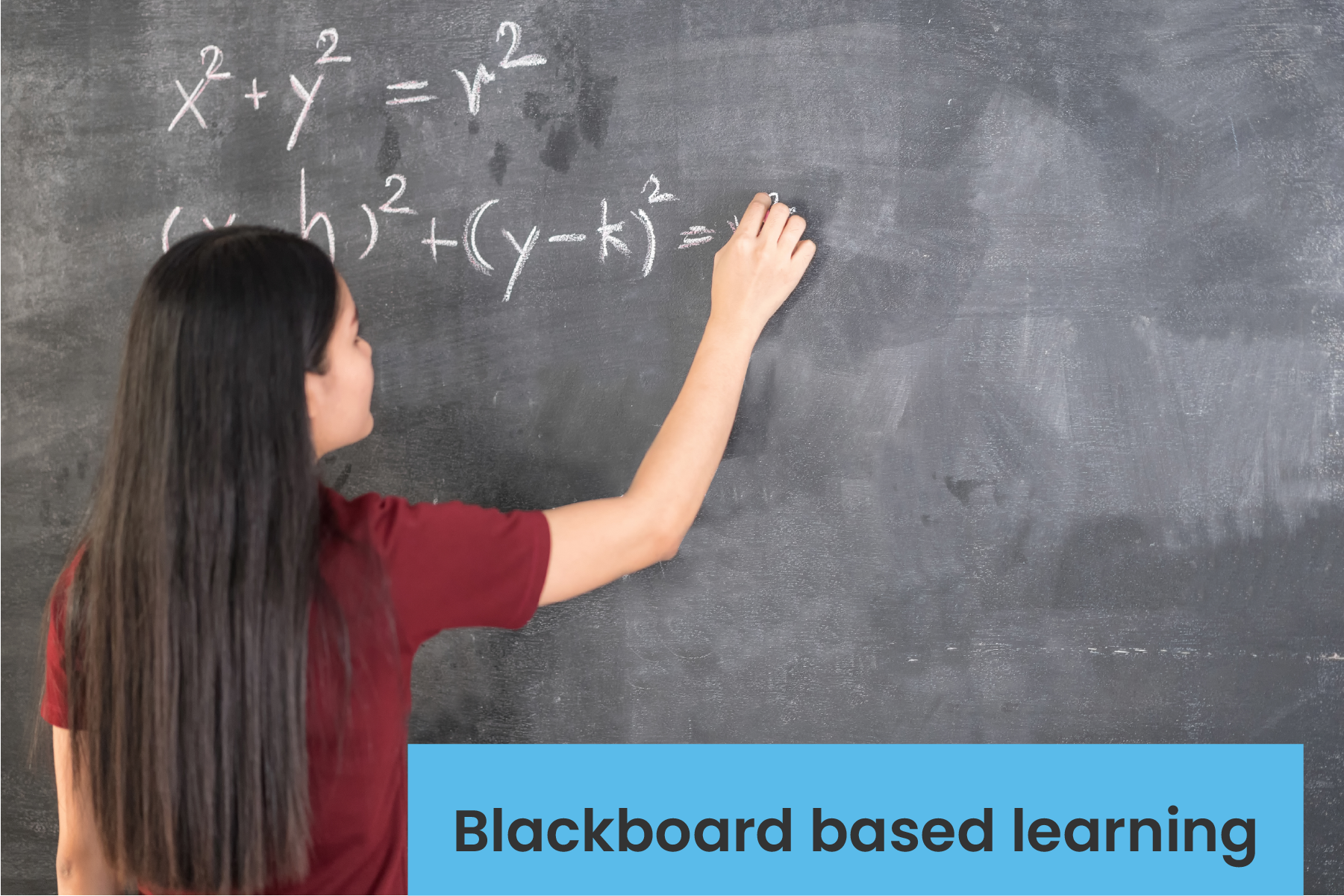Parenting

# Why is visual mathematics great for your child?

## visual mathematics

While studying about the impact of visualization on a child’s understanding of mathematics, Stanford University professor Jo Boaler noted that different sections of a child’s brain light up when they perform mathematical problem-solving. Boaler’s research also showed that when a child performs simple mathematical tasks (such as a simple multiplication of two numbers), the visual processing parts of the brain (ventral and dorsal pathways) get activated.

You’d be surprised to know, but your child consciously tries to visualize mathematical operations in their head, even for the most basic of mathematical tasks!

However, many parents still perceive mathematics as an abstract subject involving numbers and symbols. When trying to explain the importance of visual tools in teaching mathematical concepts in schools, math experts around the world have to contend with this reaction regularly – ‘But isn’t visualizing mathematical concepts only for low levels of learners?’

When your child learns through visual tools and manipulatives, the process of mathematical learning changes for them. Different international organizations for mathematics like the National Council for the Teaching of Mathematics (NCTM) and the Mathematical Association of America (MAA) have consistently advocated for the use of multiple visual representations in a student’s learning of mathematics.

But for thousands of students all across India, mathematics as a subject is still taught in a broadcast manner wherein the teacher describes the concepts on a blackboard to a class of 30 something students. Such a form of learning hinders your child from thinking visually leading to a pattern of rote learning.Moreover, the use of mathematical manipulatives or activities to promote visual information processing is something that is still yet to be entirely embraced by teachers across the country.

Such learning systems expose your child to a higher risk of developing an irrational fear of math. Recognizing these challenges, Cuemath undertook extensive research in understanding the importance of visualization, building the concept of Math Box. The Cuemath Math Box is designed to help your child visualize mathematical concepts through their senses mainly touch, feel and sight. The use of the hands to hold and move different mathematical manipulatives around increases your child’s visual-spatial understanding of the concepts.

At Cuemath, we go beyond the four walls of a classroom, helping your child understand math through visualization clubbed with practice, in a classroom focused on their overall individual visual-spatial development.

The nature of visual mathematics being much more open and creative can go a long way in reducing and eliminating the aversion, commonly seen in students towards the subject. However, the concept of using visual manipulatives as a tool to teach math to students is not readily followed in schools in India.

Math is a highly logical and objective driven subject where most of the concepts are interrelated. Technology along with other math-based manipulatives makes it easy for your child to understand concepts in a personalized way based on their capabilities.

Related Articles
GIVE YOUR CHILD THE CUEMATH EDGE
Access Personalised Math learning through interactive worksheets, gamified concepts and grade-wise courses